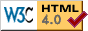## Institut für Astronomie und Astrophysik

### Abteilung Astronomie

Sand 1, D-72076 Tübingen, GermanyHinweis: Einige Seiten auf astro.uni-tuebingen.de können veraltet sein und werden nicht mehr aktualisiert.
Note: Some webpages at astro.uni-tuebingen.de may be outdated and will no longer be updated.

## Preprint 07/12

### X-ray bursting neutron star atmosphere models using an exact relativistic kinetic equation for Compton scattering

V. Suleimanov (1,2), J. Poutanen (3), K. Werner (1)

(1) Institute for Astronomy and Astrophysics, Kepler Center for Astro and Particle Physics, Eberhard Karls University, Tübingen, Germany
(2) Kazan Federal University, Russia
(3) Astronomy Division, Department of Physics, University of Oulu, Finland)

To be published in: A&A

Abstract. Theoretical spectra of X-ray bursting neutron star (NS) model atmospheres are widely used to determine the basic NS parameters such as their masses and radii. We construct accurate NS atmosphere models using for the first time an exact treatment of Compton scattering via the integral relativistic kinetic equation. We also compare the results with the previous calculations based on the Kompaneets operator. We solve the radiation transfer equation together with the hydrostatic equilibrium equation accounting exactly for the radiation pressure by electron scattering. We thus construct a new set of plane-parallel atmosphere models in LTE for hot NSs. The models were computed for six chemical compositions (pure H, pure He, solar H/He mix with various heavy elements abundances Z = 1, 0.3, 0.1, and 0.01 Zsun, and three log g = 14.0, 14.3, and 14.6. For each chemical composition and log g, we compute more than 26 model atmospheres with various luminosities relative to the Eddington luminosity LEdd computed for the Thomson cross-section. The maximum relative luminosities L/LEdd reach values of up to 1.1 for high gravity models. The emergent spectra of all models are redshifted and fitted by diluted blackbody spectra in the 3--20 keV energy range appropriate for the RXTE/PCA. We also compute the color correction factors fc. The radiative acceleration grad in our luminous, hot-atmosphere models is significantly smaller than in corresponding models based on the Kompaneets operator, because of the Klein-Nishina reduction of the electron scattering cross-section, and therefore formally "super-Eddington" model atmospheres do exist. The differences between the new and old model atmospheres are small for L / LEdd < 0.8. For the same grad / g, the new fc are slightly larger (by approximately 1%) than the old values.

Astrophysics (astro-ph): 1208.1467

 Jürgen Barnstedt (barnstedt AT astro.uni-tuebingen.de) | Impressum Last modified 13 Aug 2012